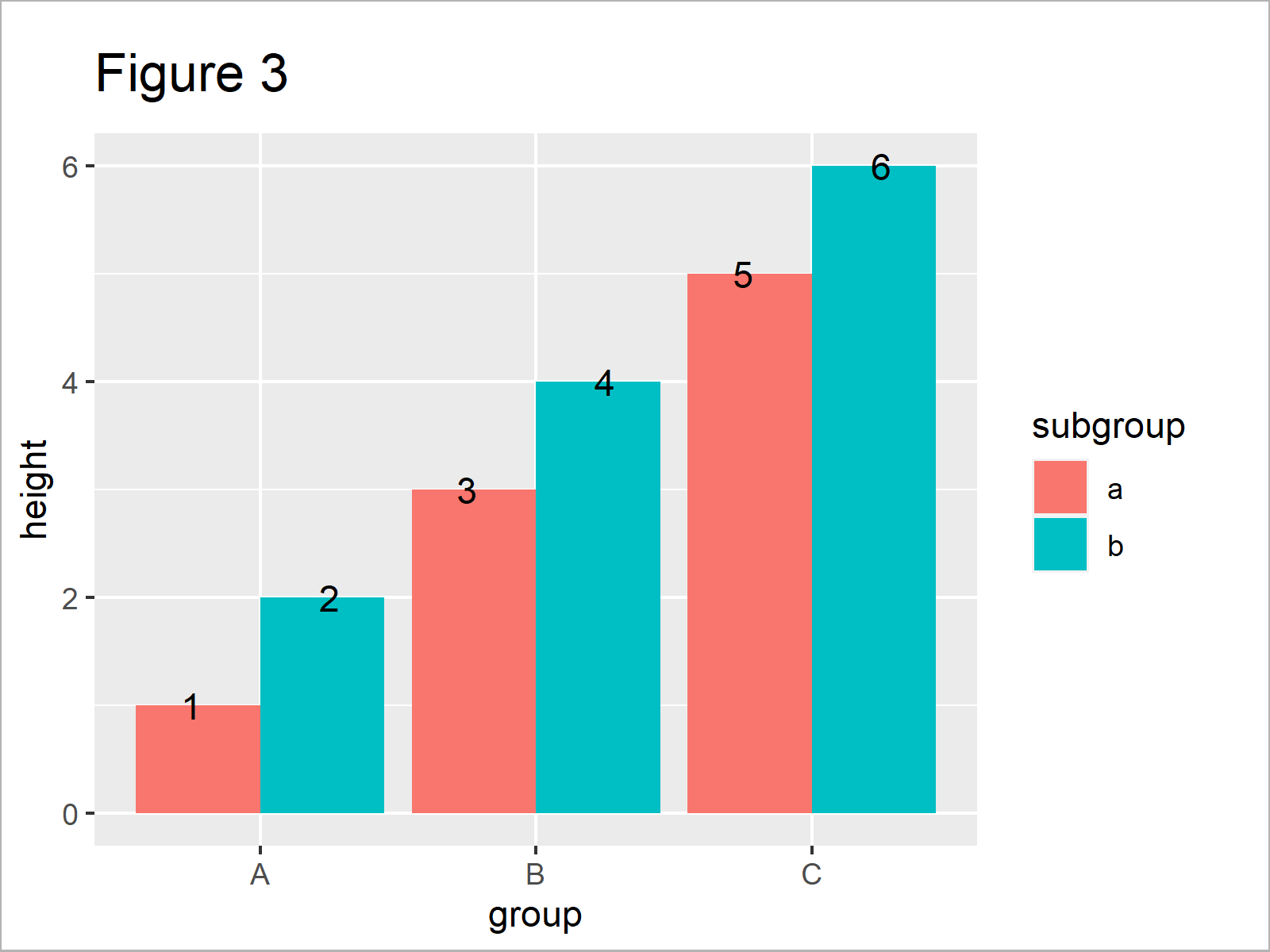# Position geom_text Labels in Grouped ggplot2 Barplot in R (Example)

In this article, I’ll demonstrate how to properly add text labels to a dodged ggplot2 barchart in R.

The article consists of these contents:

Let’s take a look at some R codes in action…

## Example Data, Packages & Basic Graphic

We’ll use the following data as basement for this R tutorial.

```data <- data.frame(height = 1:6,      # Create example data
group = rep(LETTERS[1:3], each = 2),
subgroup = letters[1:2])
data                                  # Print example data```Table 1 illustrates the output of the RStudio console returned by the previous syntax and shows that our example data is composed of six rows and three variables.

The variable height defines the heights of our bars and the variables group and subgroup define our different bars.

For the example of this tutorial, we’ll also need to install and load the ggplot2 package:

```install.packages("ggplot2")           # Install ggplot2 package

Next, we can draw the data in a grouped ggplot2 barplot:

```ggp <- ggplot(data, aes(x = group,    # Create ggplot2 plot without labels
y = height,
fill = subgroup)) +
geom_bar(stat = "identity",
position = "dodge")
ggp                                   # Draw ggplot2 plot without labels```In Figure 1 it is shown that we have plotted a grouped ggplot2 barchart with dodged positions by executing the previous syntax.

Next, we may add text labels on top of the bars using the geom_text function:

```ggp +                                 # Add text labels at wrong positions
geom_text(aes(group, label = height))```After running the previous R programming syntax the bargraph illustrated in Figure 2 has been plotted. As you can see, the text labels on top of the bars are not aligned properly.

So how can we change the positioning of our text labels? That’s what I’ll explain in the following example!

## Example: Specify Correct Text Label Positions of Dodged Barplot

This example illustrates how to add geom_text labels at the top of each bar of our grouped barplot.

For this, we have to specify the position argument within the geom_text function to be equal to position_dodge(width = 1).

Have a look at the following R code:

```ggp +                                 # Add text labels at correct positions
geom_text(aes(group, label = height),
position = position_dodge(width = 1))```In Figure 3 you can see that we have plotted a grouped barplot with properly located text counts on the of the bars using the previous code.

## Video & Further Resources

Have a look at the following video of my YouTube channel. In the video, I demonstrate the R code of this article:

Furthermore, you may read some of the other tutorials on my website. I have published numerous tutorials already.

In summary: In this article, I have demonstrated how to use the geom_text function to draw text labels on top of the bars of a grouped barplot in the R programming language. Don’t hesitate to tell me about it in the comments section, if you have additional questions.

Subscribe to the Statistics Globe Newsletter

•Marta
October 29, 2021 10:55 am

Hi Joachim,

Thank you so much for the great tutorials! I wanted to ask if it is possible to use geom_text to add labels on bars that have a different width.
The bars of my histogram each have a completely different width and I would still like to add a label in the middle of each bar.

Thank you and best!

•October 29, 2021 12:56 pm

Hey Marta,

Thanks a lot for the very kind feedback!

Regarding your question: The following code works fine for me.

```data <- data.frame(height = 1:6,      # Create example data
group = LETTERS[1:6])
data                                  # Print example data

ggp &lt;- ggplot(data, aes(x = group,    # Create ggplot2 plot without labels
y = height)) +
geom_bar(stat = "identity",
position = "dodge",
width = seq(0.1, 0.6, 0.1))
ggp                                   # Draw ggplot2 plot without labels

ggp +                                 # Add text labels at correct positions
geom_text(aes(group, label = height),
position = position_dodge(width = 1))```

Regards,
Joachim

•Will
January 28, 2022 1:13 pm

Thanks for this helpful tutorial. I have included error bars on my plot, so it makes the plotting the text hard to read and messy. Is there a way to shift the text so it sits above the error bars?

•February 7, 2022 11:49 am

Hey Will,

Regarding your question: You may use the vjust argument for this. Have a look at the example code below:

```ggp +
geom_text(aes(group, label = height),
position = position_dodge(width = 1),
vjust = - 2)```

Regards,
Joachim

•Christine
April 6, 2022 10:11 am

Thank you very much, your tutorial described exactly the problem I had with my grouped Barplot. Is there a way to place these labels at the bottom of the bars, probably on y=0.5?

•April 6, 2022 11:08 am

Hey Christine,

Thank you for the kind comment, glad it was useful!

Regards,
Joachim

•Bethany
July 27, 2022 12:42 pm

Hi this is very useful! I was just wondering if there was a way to add the text to say all the blue bars, but not the pink ones. In need of adding n= to grouped bar plot but just for one bar for each group if that makes sense!

•July 27, 2022 1:38 pm

Hey Bethany,

You could define the labels manually as shown below:

```my_height <- data\$height
my_height[c(1, 3, 5)] <- ""

ggp +
geom_text(aes(group, label = my_height),
position = position_dodge(width = 1))```Regards,
Joachim

•junru
March 7, 2023 7:43 am

I have clusters with uneven numbers of bars. To keep the bars of the same width, I specified the bars with:
geom_bar(position=position_dodge(preserve = c(“single”)), stat=”identity”,color = 1, size = 3)
and
the error_bars with:
geom_errorbar(aes(ymin= lower.CL, ymax=upper.CL),
width=.5, # Width of the error bars
size = 2,
position=position_dodge(width = 0.9,preserve = c(“single”)))
However, if I use the following code to arrange the texts, the texts are not in the right place.
geom_text(aes(x=group,label = subgroup),
vjust = 3,
position=position_dodge(width=1,preserve = c(“single”)))

•March 8, 2023 8:08 am